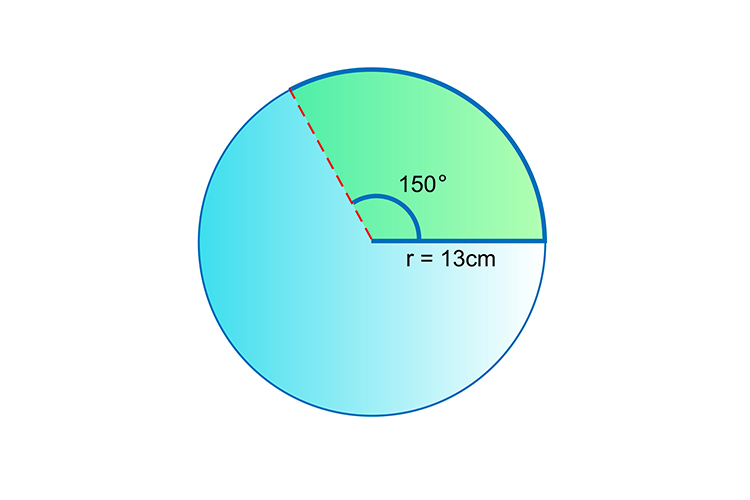# Area of a sector

Using the same reasoning about radians we can also work out the area of any sector of a circle.

Example

Work out the area of the shaded areaArea of a whole circle=pir^2

=pitimes13^2 cm^2

Logic progresses as follows:

360^@=pitimes13^2 cm^2

150^@=x cm^2

 360/150 = (pi13^2)/x x = (pi13^2times150)/360 x = 221.22...... x ~~ 221 cm^2

The area of the segment is approximately 221 cm^2